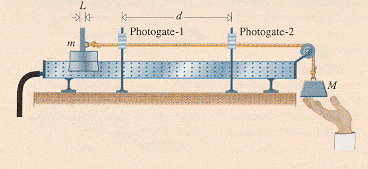# A glider on an air track

A 0.50 kg glider on an air track is pulled along frictionlessly by a tensile force arising from a hanging 19.0 N weight. Over what distance will it travel in order that 14.0 J of work be done on it?I've been set this question by one of my Physics teachers and it's got me a little confused...I conducted some research, and I think that the tensile is the force used to accelerate both masses, not just the hanging one. So do I need to find the acceleration it produces? Then I can find the force on the 0.50kg mass, and could consequently find the distance travelled from the given work done...but I don't know if this is right, and if it is, how I go about doing it! Many thanks.

Doc Al
Mentor
The tensile strength is the tension in the string. That's the only net force acting on the glider, so it's the force doing the work on the glider. To solve for the tension, apply Newton's 2nd law to each mass.

OK... so the total net force is 19N as there are no other forces acting on either of the masses. So I can apply this to the second weight:
F = ma
19 = m_2 x 9.81
m_2 = 19/19.81
m_2 = 1.93kg
Now for calculating the force for the glider do I keep a as 9.81?

If 19.0N is the total force, why can I not just use W = Fd to work out the distance travelled?

W = Fd
14 = 19 x d
d = 14 / 19
d = 0.83m

?

Doc Al
Mentor
You've calculated the mass of the hanging weight. Good. Now apply Newton's 2nd law to each mass, giving you two equations. You'll solve those equations to find the acceleration and the tension.

Doc Al
Mentor
lando45 said:
If 19.0N is the total force, why can I not just use W = Fd to work out the distance travelled?
You need the force on the glider, which is the tension in the string. You need to solve for that tension. (It's not equal to the weight of the hanging mass!)

Doc Al said:
You need the force on the glider, which is the tension in the string. You need to solve for that tension. (It's not equal to the weight of the hanging mass!)
How is it not the same as the weight of the hanging mass? I think that's what's confusing me...if the hanging mass weighs 1.93kg, then the force it exerts on the rope (i.e. the tension force) is surely just 1.93 x 9.81 which brings you back to the original 19.0N?

OK so here's what I did next...

19.0N = 0.5kg x a
a_g = 38ms^-1

19.0N = 1.93kg x a
a_h = 9.81ms^-1

Does this seem OK? And where can I take it from here? It seems anything I try just brings me back to 19.0...

Doc Al
Mentor
lando45 said:
How is it not the same as the weight of the hanging mass? I think that's what's confusing me...if the hanging mass weighs 1.93kg, then the force it exerts on the rope (i.e. the tension force) is surely just 1.93 x 9.81 which brings you back to the original 19.0N?
Not true. If the string tension equaled the weight of the hanging mass, then the mass would be in equilibrium and it wouldn't fall.

Instead, analyze the forces on both masses: On the hanging mass, tension pulls up, gravity pulls down. The net force leads to some downward acceleration. (Note that the acceleration is not 9.8 m/s^2 -- that's the acceleration of an object in free fall, where gravity is the only force acting.)